Next: 5.3.4 Cosine Distribution Up: 5.3 Generation of Random Previous: 5.3.2 Coned Cosine Distribution

## 5.3.3 Direction Vector Calculation

Ray tracing usually requires a normalized direction vector. Therefore, once random polar and azimuthal angles with respect to the given unit vector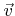are determined, the direction vector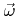can be obtained by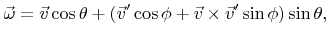(5.49)

where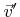is a normal vector with respect to, thus satisfying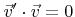. The rotational symmetry of the random direction distribution allows an arbitrary choice of, which can be defined as follows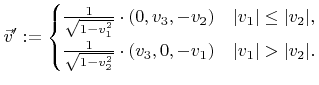(5.50)

The case differentiation avoids problematic cases, wherevanishes. In the following, only the first case is considered. The second case can be converted to the first case by exchanging the first two components of,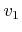and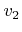, before calculating. A final exchange of the corresponding components of,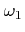and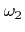, leads to the correct direction vector.

The condition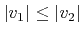together with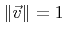implies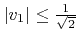and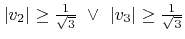. Therefore,is always well-defined. Insertion into (5.49) gives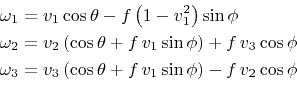with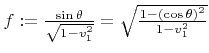. Using the second notation of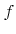,can be calculated without knowledge of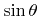at the expense of one additional multiplication. Some distributions, such as the previously discussed power cosine distribution, enable the direct calculation of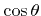, avoiding the costly evaluation of trigonometric functions for the polar angle entirely (see the last note in Algorithm 5.2).

The evaluation of the other trigonometric functions in (5.51) can also be avoided. The pointis uniformly distributed on the unit circle. An alternative way for picking a point on the unit circle is to randomly choose a point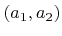on a disk and to calculate the normalized vector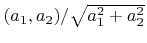. The radicand can be combined with that for the calculation ofobviating the extra evaluation of the root. Algorithm 5.4 describes the determination of a random unit vectoraroundwith given polar angle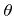, which is equivalent to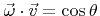.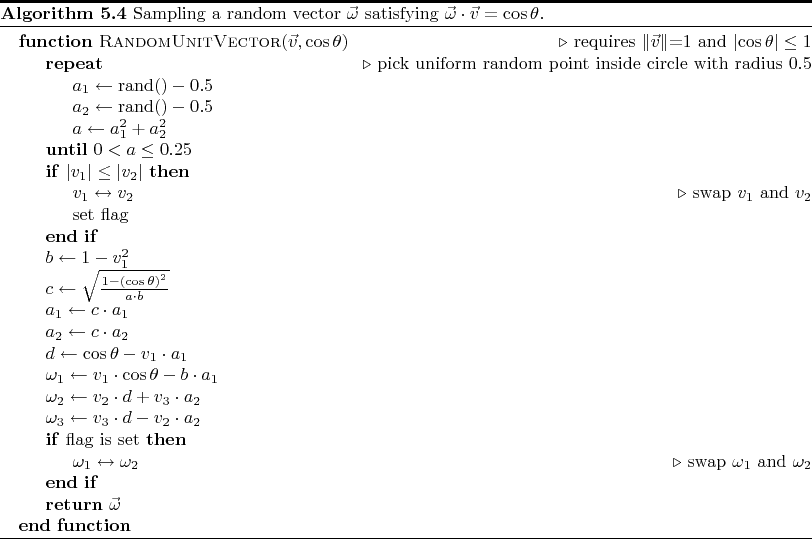Next: 5.3.4 Cosine Distribution Up: 5.3 Generation of Random Previous: 5.3.2 Coned Cosine Distribution

Otmar Ertl: Numerical Methods for Topography Simulation